HomeCompositio Mathematica

# Lattice structure of Weyl groups via representation theory of preprojective algebras

Published online by Cambridge University Press:  16 May 2018

Corresponding

## Abstract

This paper studies the combinatorics of lattice congruences of the weak order on a finite Weyl group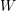$W$ , using representation theory of the corresponding preprojective algebra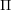$\unicode[STIX]{x1D6F1}$ . Natural bijections are constructed between important objects including join-irreducible congruences, join-irreducible (respectively, meet-irreducible) elements of$W$ , indecomposable$\unicode[STIX]{x1D70F}$ -rigid (respectively,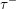$\unicode[STIX]{x1D70F}^{-}$ -rigid) modules and layers of$\unicode[STIX]{x1D6F1}$ . The lattice-theoretically natural labelling of the Hasse quiver by join-irreducible elements of$W$ is shown to coincide with the algebraically natural labelling by layers of$\unicode[STIX]{x1D6F1}$ . We show that layers of$\unicode[STIX]{x1D6F1}$ are nothing but bricks (or equivalently stones, or 2-spherical modules). The forcing order on join-irreducible elements of$W$ (arising from the study of lattice congruences) is described algebraically in terms of the doubleton extension order. We give a combinatorial description of indecomposable$\unicode[STIX]{x1D70F}^{-}$ -rigid modules for type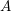$A$ and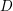$D$ .

## MSC classification

Type
Research Article
Information
Compositio Mathematica , June 2018 , pp. 1269 - 1305

## Access options

Get access to the full version of this content by using one of the access options below.

## References

Adachi, T., Iyama, O. and Reiten, I., 𝜏-tilting theory , Compos. Math. 150 (2014), 415452.CrossRefGoogle Scholar
Aihara, T. and Mizuno, Y., Classifying tilting complexes over preprojective algebras of Dynkin type , Algebra Number Theory 11 (2017), 12871315.CrossRefGoogle Scholar
Amiot, C., Cluster categories for algebras of global dimension 2 and quivers with potential , Ann. Inst. Fourier (Grenoble) 59 (2009), 25252590.CrossRefGoogle Scholar
Amiot, C., Iyama, O., Reiten, I. and Todorov, G., Preprojective algebras and c-sortable words , Proc. Lond. Math. Soc. (3) 104 (2012), 513539.CrossRefGoogle Scholar
Auslander, M. and Smalo, S. O., Almost split sequences in subcategories , J. Algebra 69 (1981), 426454.CrossRefGoogle Scholar
Baer, D., Geigle, W. and Lenzing, H., The preprojective algebra of a tame hereditary Artin algebra , Comm. Algebra 15 (1987), 425457.CrossRefGoogle Scholar
Baumann, P., Kamnitzer, J. and Tingley, P., Affine Mirković–Vilonen polytopes , Publ. Math. Inst. Hautes Études Sci. 120 (2014), 113205.CrossRefGoogle Scholar
Björner, A. and Brenti, F., Combinatorics of Coxeter groups, Graduate Texts in Mathematics, vol. 231 (Springer, New York, 2005).Google Scholar
Bolten, B., Spiegelungsfunktoren für präprojektive Algebren, Diplomarbeit, Bonn (2010).Google Scholar
Bongartz, J., Endotrivial modules over preprojective algebras, Master’s thesis, Bonn (2015).Google Scholar
Buan, A., Iyama, O., Reiten, I. and Scott, J., Cluster structures for 2-Calabi–Yau categories and unipotent groups , Compos. Math. 145 (2009), 10351079.CrossRefGoogle Scholar
Caspard, N., Le Conte de Poly-Barbut, C. and Morvan, M., Cayley lattices of finite Coxeter groups are bounded , Adv. Appl. Math. 33 (2004), 7194.CrossRefGoogle Scholar
Crawley-Boevey, W. and Holland, M. P., Noncommutative deformations of Kleinian singularities , Duke Math. J. 92 (1998), 605635.CrossRefGoogle Scholar
Day, A., Congruence normality: the characterization of the doubling class of convex sets , Algebra Universalis 31 (1994), 397406.CrossRefGoogle Scholar
Demonet, L., Iyama, O. and Jasso, G.,$\unicode[STIX]{x1D70F}$ -tilting finite algebras, bricks and$g$ -vectors, Int. Math. Res. Not. IMRN, to appear. Preprint (2015), arXiv:1503.00285.Google Scholar
Demonet, L., Iyama, O., Reiten, I., Reading, N. and Thomas, H., Lattice theory of torsion classes, Preprint (2017), arXiv:1711.01785.Google Scholar
Dlab, V. and Ringel, C. M., The preprojective algebra of a modulated graph , in Representation theory, II (Proc. Second Internat. Conf., Carleton Univ., Ottawa, Ont., 1979), Lecture Notes in Mathematics, vol. 832 (Springer, Berlin, New York, 1980), 216231.Google Scholar
Geiss, C., Leclerc, B. and Schröer, J., Kac-Moody groups and cluster algebras , Adv. Math. 228 (2011), 329433.CrossRefGoogle Scholar
Happel, D., Hartlieb, S., Kerner, O. and Unger, L., On perpendicular categories of stones over quiver algebras , Comment. Math. Helv. 71 (1996), 463474.CrossRefGoogle Scholar
Iyama, O. and Reiten, I., Fomin–Zelevinsky mutation and tilting modules over Calabi–Yau algebras , Amer. J. Math. 130 (2008), 10871149.CrossRefGoogle Scholar
Iyama, O., Reiten, I., Thomas, H. and Todorov, G., Lattice structure of torsion classes for path algebras , Bull. Lond. Math. Soc. 47 (2015), 639650.CrossRefGoogle Scholar
Kashiwara, M. and Saito, Y., Geometric construction of crystal bases , Duke Math. J. 89 (1997), 936.CrossRefGoogle Scholar
Keller, B., Calabi–Yau triangulated categories , in Trends in representation theory of algebras and related topics, EMS Ser. Congr. Rep. (Eur. Math. Soc., Zürich, 2008), 467489.CrossRefGoogle Scholar
Kerner, O. and Lukas, F., Regular stones of wild hereditary algebras , J. Pure Appl. Algebra 93 (1994), 1531.CrossRefGoogle Scholar
Kimura, Y., Tilting theory of preprojective algebras and c-sortable elements , J. Algebra 503 (2018), 186221.CrossRefGoogle Scholar
Leclerc, B., Cluster structures on strata of flag varieties , Adv. Math. 300 (2016), 190228.CrossRefGoogle Scholar
Lusztig, G., Quivers, perverse sheaves, and quantized enveloping algebras , J. Amer. Math. Soc. 4 (1991), 365421.CrossRefGoogle Scholar
Marks, F., Homological embeddings for preprojective algebras , Math. Z. 285 (2017), 10911106.CrossRefGoogle Scholar
Mizuno, Y., Classifying 𝜏-tilting modules over preprojective algebras of Dynkin type , Math. Z. 277 (2014), 665690.CrossRefGoogle Scholar
Nakajima, H., Instantons on ALE spaces, quiver varieties, and Kac–Moody algebras , Duke Math. J. 76 (1994), 365416.CrossRefGoogle Scholar
Oppermann, S., Reiten, I. and Thomas, H., Quotient closed subcategories of quiver representations , Compos. Math. 151 (2015), 568602.CrossRefGoogle Scholar
Reading, N., Lattice congruences of the weak order , Order 21 (2004), 315344.CrossRefGoogle Scholar
Reading, N., Lattice theory of the poset of regions , in Lattice theory: special topics and applications, Vol. 2 (Birkhäuser/Springer, Cham, 2016), 399487.CrossRefGoogle Scholar
Reading, N., Finite Coxeter groups and the weak order , in Lattice theory: special topics and applications, Vol. 2 (Birkhäuser/Springer, Cham, 2016), 489561.CrossRefGoogle Scholar
Ringel, C. M., Representations of K-species and bimodules , J. Algebra 41 (1976), 269302.CrossRefGoogle Scholar
Smalø, S. O., Torsion theories and tilting modules , Bull. Lond. Math. Soc. 16 (1984), 518522.CrossRefGoogle Scholar
Seidel, P. and Thomas, R., Braid group actions on derived categories of coherent sheaves , Duke Math. J 108 (2001), 37108.Google Scholar
Sekiya, Y. and Yamaura, K., Tilting theoretical approach to moduli spaces over preprojective algebras , Algebr. Represent. Theory 16 (2013), 17331786.CrossRefGoogle Scholar
Wald, B. and Waschbüsch, J., Tame biserial algebras , J. Algebra 95 (1985), 480500.CrossRefGoogle Scholar

### Full text views

Full text views reflects PDF downloads, PDFs sent to Google Drive, Dropbox and Kindle and HTML full text views.

Total number of HTML views: 4
Total number of PDF views: 141 *
View data table for this chart

* Views captured on Cambridge Core between 16th May 2018 - 18th January 2021. This data will be updated every 24 hours.

Hostname: page-component-77fc7d77f9-w9qs9 Total loading time: 0.344 Render date: 2021-01-18T08:27:33.706Z Query parameters: { "hasAccess": "0", "openAccess": "0", "isLogged": "0", "lang": "en" } Feature Flags last update: Mon Jan 18 2021 08:02:51 GMT+0000 (Coordinated Universal Time) Feature Flags: { "metrics": true, "metricsAbstractViews": false, "peerReview": true, "crossMark": true, "comments": true, "relatedCommentaries": true, "subject": true, "clr": true, "languageSwitch": true, "figures": false, "newCiteModal": false, "shouldUseShareProductTool": true, "shouldUseHypothesis": true, "isUnsiloEnabled": true }

# Send article to Kindle

Note you can select to send to either the @free.kindle.com or @kindle.com variations. ‘@free.kindle.com’ emails are free but can only be sent to your device when it is connected to wi-fi. ‘@kindle.com’ emails can be delivered even when you are not connected to wi-fi, but note that service fees apply.

Find out more about the Kindle Personal Document Service.

Lattice structure of Weyl groups via representation theory of preprojective algebras
Available formats
×

# Send article to Dropbox

To send this article to your Dropbox account, please select one or more formats and confirm that you agree to abide by our usage policies. If this is the first time you use this feature, you will be asked to authorise Cambridge Core to connect with your <service> account. Find out more about sending content to Dropbox.

Lattice structure of Weyl groups via representation theory of preprojective algebras
Available formats
×

# Send article to Google Drive

To send this article to your Google Drive account, please select one or more formats and confirm that you agree to abide by our usage policies. If this is the first time you use this feature, you will be asked to authorise Cambridge Core to connect with your <service> account. Find out more about sending content to Google Drive.

Lattice structure of Weyl groups via representation theory of preprojective algebras
Available formats
×
×